# Python | NLP analysis of Restaurant reviews

• Difficulty Level : Easy
• Last Updated : 02 Nov, 2021

Natural language processing (NLP) is an area of computer science and artificial intelligence concerned with the interactions between computers and human (natural) languages, in particular how to program computers to process and analyze large amounts of natural language data. It is the branch of machine learning which is about analyzing any text and handling predictive analysis.
Scikit-learn is a free software machine learning library for the Python programming language. Scikit-learn is largely written in Python, with some core algorithms written in Cython to achieve performance. Cython is a superset of the Python programming language, designed to give C-like performance with code that is written mostly in Python.
Let’s understand the various steps involved in text processing and the flow of NLP.
This algorithm can be easily applied to any other kind of text like classify a book into Romance, Friction, but for now, let’s use a restaurant review dataset to review negative or positive feedback.

#### Steps involved:

Step 1: Import dataset with setting delimiter as ‘\t’ as columns are separated as tab space. Reviews and their category(0 or 1) are not separated by any other symbol but with tab space as most of the other symbols are is the review (like \$ for the price, ….!, etc) and the algorithm might use them as a delimiter, which will lead to strange behavior (like errors, weird output) in output.

## Python3

 `# Importing Libraries` `import` `numpy as np  ` `import` `pandas as pd `   `# Import dataset` `dataset ``=` `pd.read_csv(``'Restaurant_Reviews.tsv'``, delimiter ``=` `'\t'``) `

Step 2: Text Cleaning or Preprocessing

• Remove Punctuations, Numbers: Punctuations, Numbers don’t help much in processing the given text, if included, they will just increase the size of a bag of words that we will create as the last step and decrease the efficiency of an algorithm.
• Stemming: Take roots of the word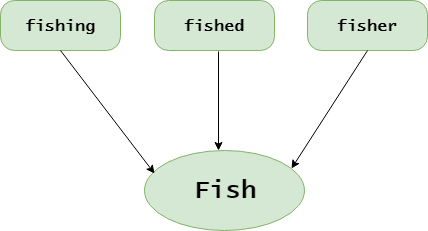• Convert each word into its lower case: For example, it is useless to have some words in different cases (eg ‘good’ and ‘GOOD’).

## Python3

 `# library to clean data` `import` `re `   `# Natural Language Tool Kit` `import` `nltk `   `nltk.download(``'stopwords'``)`   `# to remove stopword` `from` `nltk.corpus ``import` `stopwords`   `# for Stemming propose ` `from` `nltk.stem.porter ``import` `PorterStemmer`   `# Initialize empty array` `# to append clean text ` `corpus ``=` `[] `   `# 1000 (reviews) rows to clean` `for` `i ``in` `range``(``0``, ``1000``): ` `    `  `    ``# column : "Review", row ith` `    ``review ``=` `re.sub(``'[^a-zA-Z]'``, ``' '``, dataset[``'Review'``][i]) ` `    `  `    ``# convert all cases to lower cases` `    ``review ``=` `review.lower() ` `    `  `    ``# split to array(default delimiter is " ")` `    ``review ``=` `review.split() ` `    `  `    ``# creating PorterStemmer object to` `    ``# take main stem of each word` `    ``ps ``=` `PorterStemmer() ` `    `  `    ``# loop for stemming each word` `    ``# in string array at ith row    ` `    ``review ``=` `[ps.stem(word) ``for` `word ``in` `review` `                ``if` `not` `word ``in` `set``(stopwords.words(``'english'``))] ` `                `  `    ``# rejoin all string array elements` `    ``# to create back into a string` `    ``review ``=` `' '``.join(review)  ` `    `  `    ``# append each string to create` `    ``# array of clean text ` `    ``corpus.append(review) `

Examples: Before and after applying above code (reviews = > before, corpus => after)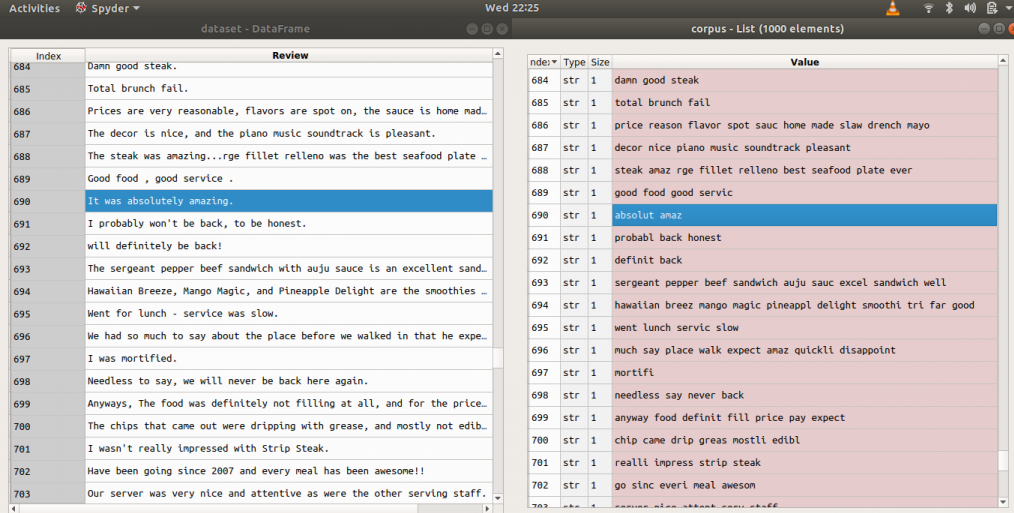Step 3: Tokenization, involves splitting sentences and words from the body of the text.
Step 4: Making the bag of words via sparse matrix

• Take all the different words of reviews in the dataset without repeating of words.
• One column for each word, therefore there is going to be many columns.
• Rows are reviews
• If a word is there in the row of a dataset of reviews, then the count of the word will be there in the row of a bag of words under the column of the word.

Examples: Let’s take a dataset of reviews of only two reviews

```Input : "dam good steak", "good food good service"
Output :
```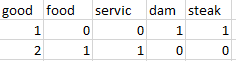` `

For this purpose we need CountVectorizer class from sklearn.feature_extraction.text.
We can also set a max number of features (max no. features which help the most via attribute “max_features”). Do the training on the corpus and then apply the same transformation to the corpus “.fit_transform(corpus)” and then convert it into an array. If the review is positive or negative that answer is in the second column of the dataset[:, 1]: all rows and 1st column (indexing from zero).

## Python3

 `# Creating the Bag of Words model` `from` `sklearn.feature_extraction.text ``import` `CountVectorizer`   `# To extract max 1500 feature.` `# "max_features" is attribute to` `# experiment with to get better results` `cv ``=` `CountVectorizer(max_features ``=` `1500``) `   `# X contains corpus (dependent variable)` `X ``=` `cv.fit_transform(corpus).toarray() `   `# y contains answers if review` `# is positive or negative` `y ``=` `dataset.iloc[:, ``1``].values `

Description of the dataset to be used:

• Columns separated by \t (tab space)
• First column is about reviews of people
• In second column, 0 is for negative review and 1 is for positive review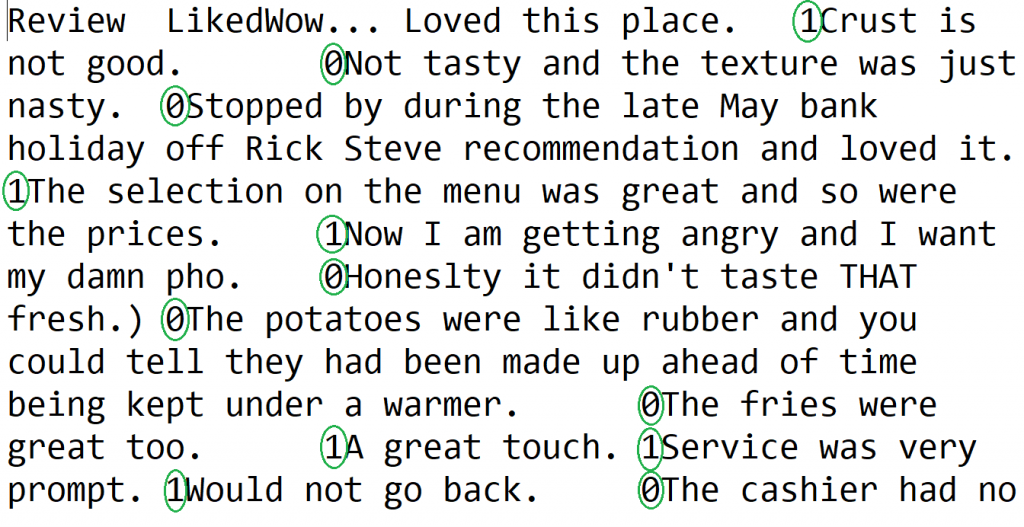Step 5: Splitting Corpus into Training and Test set. For this, we need class train_test_split from sklearn.cross_validation. Split can be made 70/30 or 80/20 or 85/15 or 75/25, here I choose 75/25 via “test_size”.
X is the bag of words, y is 0 or 1 (positive or negative).

## Python3

 `# Splitting the dataset into` `# the Training set and Test set` `from` `sklearn.cross_validation ``import` `train_test_split`   `# experiment with "test_size"` `# to get better results` `X_train, X_test, y_train, y_test ``=` `train_test_split(X, y, test_size ``=` `0.25``)`

Step 6: Fitting a Predictive Model (here random forest)

• Since Random forest is an ensemble model (made of many trees) from sklearn.ensemble, import RandomForestClassifier class
• With 501 trees or “n_estimators” and criterion as ‘entropy’
• Fit the model via .fit() method with attributes X_train and y_train

## Python3

 `# Fitting Random Forest Classification` `# to the Training set` `from` `sklearn.ensemble ``import` `RandomForestClassifier`   `# n_estimators can be said as number of` `# trees, experiment with n_estimators` `# to get better results ` `model ``=` `RandomForestClassifier(n_estimators ``=` `501``,` `                            ``criterion ``=` `'entropy'``)` `                            `  `model.fit(X_train, y_train) `

Step 7: Predicting Final Results via using .predict() method with attribute X_test

## Python3

 `# Predicting the Test set results` `y_pred ``=` `model.predict(X_test)`   `y_pred`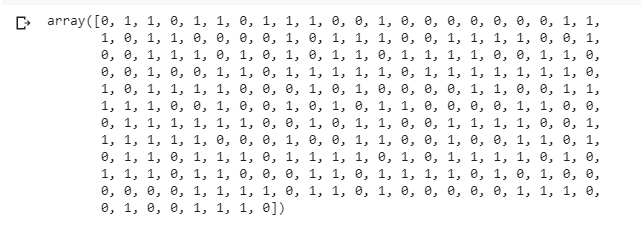Note: Accuracy with the random forest was 72%.(It may be different when performed an experiment with different test sizes, here = 0.25).
Step 8: To know the accuracy, a confusion matrix is needed.
Confusion Matrix is a 2X2 Matrix.

TRUE POSITIVE : measures the proportion of actual positives that are correctly identified.
TRUE NEGATIVE : measures the proportion of actual positives that are not correctly identified.
FALSE POSITIVE : measures the proportion of actual negatives that are correctly identified.
FALSE NEGATIVE : measures the proportion of actual negatives that are not correctly identified.

Note: True or False refers to the assigned classification being Correct or Incorrect, while Positive or Negative refers to assignment to the Positive or the Negative Category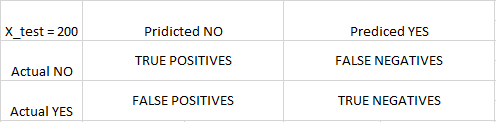## Python3

 `# Making the Confusion Matrix` `from` `sklearn.metrics ``import` `confusion_matrix`   `cm ``=` `confusion_matrix(y_test, y_pred)`   `cm`My Personal Notes arrow_drop_up
Recommended Articles
Page :